Wednesday 21st October 2020CBSE Guess > Papers > Important Questions > Class XII > 2009 > Maths > Mathematics By Anil Kumar Tondak

CBSE CLASS XII

Inverse Trigonometric Functions

Q. 1. Find the value of: tan-1 (1) + cos-1 (-1/2) + sin-1 (-1/2).
Q. 2. Prove: tan-1x + tan-1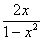= tan-1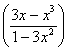, |x|<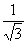Q. 3. If tan-1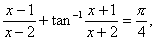then find the value of x.

Q. 4. Find the value of sin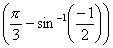.

Q. 5. Prove: sin-1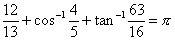Q. 6. Solve: tan-12x + tan-13x =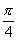Q. 7. Prove: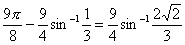Q. 8. Solve: sin-1 ( 1 –x) – 2sin-1x =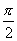.
Q. 9. Evaluate: tan-1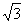- sec-1 (-2) + cosec-1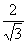.
Q. 10. Prove : tan-1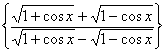=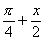Q. 11. Simplify: sin-1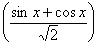,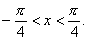Q. 12. Prove:sec2 (tan-12) + cosec2 ( cot-13) = 15.

Q. 13. Simplify : tan-1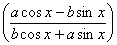Q. 14. Prove: tan-1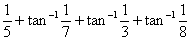=Q. 15. If sin(sin-1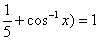, then find the value of x.

Q. 16. Prove that :
2tan-1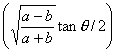= cos-1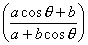Prepared By Anil Kumar Tondak
M.Sc.(Maths), M.Ed., M.A.(Eco),M.B.A.(Mkt)
Mob No. 9811363962

 Maths